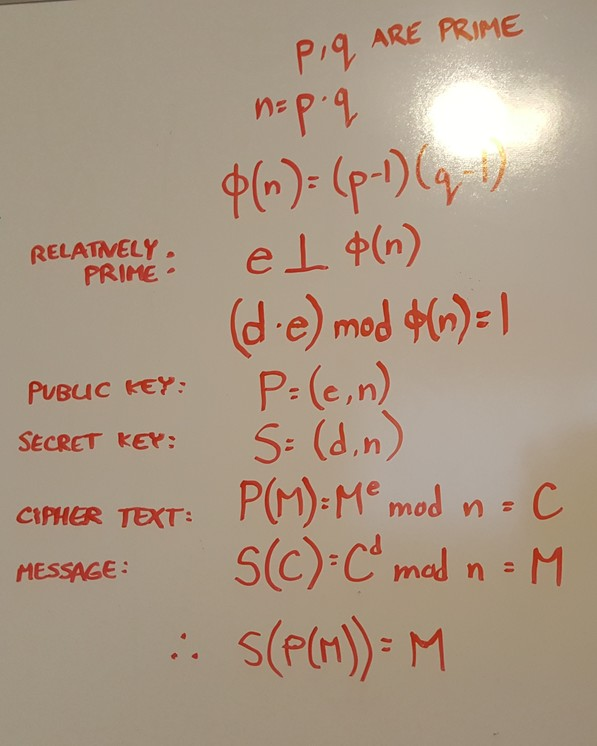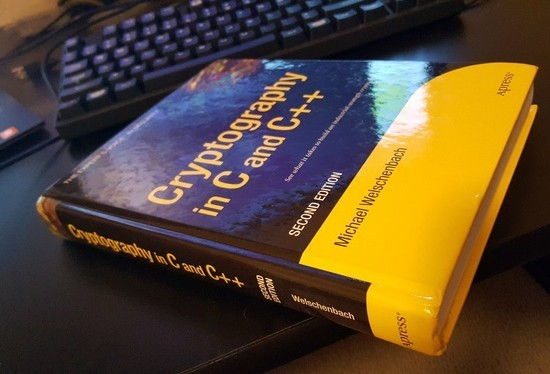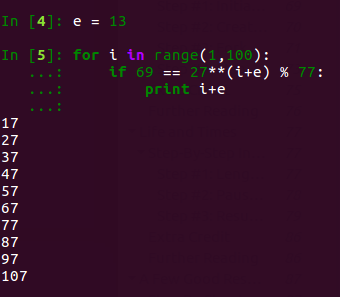In my boredom, I was exercising manual pen/paper RSA cryptography. I was calculating public/private key pairs (with very small prime numbers) and calculating the “d” (inverse e modulo t) without the use of the Extended Euclidean Algorithm (hereinafter referred to as EEA at my discretion).In the process, I thought I had made a discovery. Silly me. (Mathematicians, don’t laugh) While trial/erroring a valid “d”, my “n” value (nearly prime aka RSA modulus) and my “e” value (derived number aka relative prime to “t”) kept calculating to valid values of “d” satisfying the inverse modulo of “t”. Since brute-forcing takes so long, and I wanted to avoid the EEA, I figured, why not make an educated guess and take the difference ‘x’ of ‘n’ and ‘t’ and then add ‘x’ to ‘n’ and check to see if that is a valid ‘d’.

In other words, this was my short-lived postulate:$d = |n - t| + \begin{cases}n, & \text{if }n > t \\ t, & \text{if }n < t \end{cases}$

Well, it worked twice. Take for example:
p=3
q=5
n=15
t=8
e=7$d \in \{7,15,23\}$

or this example:
p=5
q=7
n=35
t=24
e=11$d \in \{11,35,59\}$

On the third try, I fell flat on my face and realized how silly I was:Well, technically, it does work if you substitute n and t for d1 and d2 where di is a valid d… but I’m just rediscovering what better mathematicians already know.

Now, you may be wondering why I was exercising manual RSA cryptography.
In light of recent events, I have heard lots of questions and doubts regarding the security of RSA cryptosystems, signed messages, DKIM verification, etc. I wanted to understand the cryptosystem thoroughly, look for weaknesses, and finally address these concerns.

## RSA Cryptosystem:First, we find two prime numbers p and q. The bigger the number, the harder our cryptosystem will be to decrypt. For our example, we will use something small.
Let’s say:
p=7
q=11
Then we calculate n which is merely the product of p and q. n is a “nearly prime number” because it only has 2 factors greater than 1: p and q.
n=77
Next we calculate t. t is the product of p-1 and q-1. t=(p-1)(q-1)
t=60
Next, we find a value, e (derived number). e is any number greater than 1 and less than t. e also cannot share a common factor with t besides 1. This makes e “co-prime” to t. 13 qualifies. Therefore:
e=13
Next we find d. d is the inverse of e modulo (p – 1)(q – 1) referred to as t. In other words, e * d = 1 mod t. In even other words, (d * e) / t = q remainder 1 such that q is an integer. We find:
d=37While manually calculating this (and avoiding EEA), I’ve come up with this solution to find d:$d = \frac{(t \times x)+1}{e}\ \ni\ d\in\mathbb{N}$

We now have everything we need to compose a public key and private key. The public key is (n, e) while the private key is (n, d)
public key = (77, 13)
private key = (77, 37)

Now, how does one apply this? Let’s put the algorithmic portion of this cryptosystem aside while I demonstrate with an example. I will resume the encryption/decryption portion of this after the illustration.

This is called public key encryption or asymmetric encryption because you encrypt with the public key but you can only decrypt with the private key. In Symmetric cryptosystems, the same key is used to encrypt and decrypt.

In our example, we shall use three fictional characters: Seth, Julian, and Eric. Seth has never met Julian before but wishes to pass data to him without allowing Eric’s prying eyes to read the contents of the message. Julian also wishes to allow other strangers to send him private messages in confidence with rest assurance that the message will not be read by anyone besides the intended recipient, Julian.
Julian generates a public key and a private key. He keeps the private key secret and doesn’t allow anybody to see it. He distributes the public key to the public.
Seth encrypts his confidential message with Julian’s public key. Eric cannot decrypt the message with the public key. Nobody can. Not even Seth. Once the message reaches Julian, Julian is able to decrypt the message with the private key.

Encryption of the message M and converting it into a cipher message C works like this:
C = Me mod n (since e and n are components of the public key)
let’s say we are trying to encrypt a single letter, capital ‘E’. In ASCII, this would be represented by the integer 69. So, our M is 69. Plugging that in our encryption formula, we have:
C = 6913 mod 77.
C = 803596764671634487466709 mod 77
quotient = 803596764671634487466709 / 77 = 10436321619112136200866 R 27
C = 27

Decryption of the cipher message C and converting it into a plain text message M works like this:
M = Cd mod n (since e and n are components of the private key)
M = 2737 mod 77
M = 2467502207165232736732864317691635448523249762159760 mod 77
quotient = 2467502207165232736732864317691635448523249762159760 / 77 = 32045483209938087490037198931060200630172074833243 R 69
M = 69

In case you want to try this out for yourselves, you may fall into the same trap I fell into. Be aware that your M must be smaller than n, because the modulo deals with the remainder which implies that it is smaller than n. If M is larger than n, your decrypted value M will yield a M – (n*x) where x is any integer > 1. As I write this, I realize I can further enhance this “rule” of mine:

If M is larger than n,$M' = M - n \times \lfloor M / n \rfloor$ where M’ is the decrypted M.

See? I’m not a complete idiot. Even in my blunders, I yield postulates. 🙂 In this case, I was going to use capital ‘Z’ as my plaintext message example where the ascii value was 90. Since 90 > 77, my M’ ended up being 13.

We have completed the manual process of generating public / private keys, encrypting a message, and decrypting a cipher.
You may or may not have noticed that even though the public key and private key are different, they are very similar. What is truly the difference between (n,e) and (n,d)? We know that e*d = 1 mod t. e*d = d*e. Doesn’t that mean that the public key is fully capable of switching roles with the private key and can be used to decrypt messages encrypted with the public key? Why, yes. Yes it does. As a matter of fact, that’s exactly what’s happening when messages are signed.

In our illustration above, if Julian wanted to send a message out to his readers, he could sign his message by encrypting it with the private key. The reader could then decrypt it with the public key and know for sure that the message was signed by Julian and not tampered with. Of course, in order to keep that cipher text shorter, it is more common to sign the hash of the message rather than the message itself. That way, the reader could hash the message and compare that hash with the decrypted signature.

The process I have just described is used in the DKIM verification process. When an email is DKIM verified, it is verified using the MTA’s public key to ensure that the message has not been altered nor tampered with.

Now let’s try to crack it.

## Cracking RSA

Let’s look for an attack vector. In our illustration, let’s assume we are Eric. We want to decipher Seth’s message. What we have is the C (cipher text) and the public key (n,e).

C = 27
Public Key = (77,13)
There are two vectors of attack that I could think of:

– The first and most obvious approach is to find the two prime factors of n, then calculate t and find d.

Now hold that thought and allow me to digress for a second.
Prime numbers fascinate me. The more I read about them, the more intrigued I get.I am well-aware that the biggest mathematical discovery pertaining to prime numbers was the Riemann Hypothesis which simply allows us to determine the number or prime numbers that exist below a given integer. Everything else, whether it’s the Sieve of Eratosthenes, computations used to determine Mersenne primes, etc all rely on some sort of brute-forcing.

Returning to our topic, in our simple illustration, we’re dealing with tiny 1 and 2 digit prime numbers, so we can crack our own private key by hand. n = 77. The prime factors are 7 and 11. Easy.

So, assume we developed a prime number generator and brute-forced long division against our nearly prime ‘n’.
I once wrote a prime number generator in C because a co-worker buddy challenged me to see whose generator could run faster. Mine won. Even so, a simpleton like me did not utilize the Rabin-Miller or Lehmann primality tests. It was elementary:

#include <stdio.h>
#include <stdlib.h>
#include <math.h>
#include <limits.h>

/*compile with "-std=c99" "-lm" */

unsigned long long int primes(int size){
int i=4;
int j,pass,sqrtoftest;
unsigned long long int prime[size],test;
prime=2;
prime=3;
prime=5;
prime=7;
test = 9;

while(i<=size){
pass=0;
while(!pass){
pass=1;
j=0;
test+=2;
sqrtoftest = (int) floor(sqrt(test));
while(prime[j] <= sqrtoftest){ /* check sqrt of test because the divisor should never exceed that */
if(test % prime[j] == 0){
pass=0;
break;
}
j++;
}
}
prime[i]=test;
i++;
}

return prime[size-1];
}

int main (int argc, char *argv[]){
printf("%llu\n",primes(atoi(argv)));
return 0;
}


My code was faster than my friend’s because it took advantage of a couple of shortcuts. One, I’m only dividing by the prime numbers which I have discovered in previous iterations. Two, I don’t divide by prime numbers that are larger than$\lfloor\sqrt{n}\rfloor$ where n is the number being tested for primality. Nevertheless, this code has several limitations. First of all, it’s limited to the upper bounds of an unsigned long long. This simple test will show us what the upper bound is:

#include <stdio.h>
#include <limits.h>

int main(int argc, char *argv[]){
unsigned long long x = (unsigned long long) -1;
printf("%llu\n", x);
unsigned long long y = ULLONG_MAX;
printf("%llu\n", y);
return 0;
}


On my 64-bit Ubuntu Linux laptop, it prints:
18446744073709551615
18446744073709551615

That’s the largest integer I can calculate with the built in types as verified by the “-1” test and the ULLONG_MAX macro test as provided by limits.h on a 64-bit GNU-C compiler. That’s 20 digits long. The current RSA standard is 2048-bits, which is 617 decimal digits… so we’re not even close. If processors became so powerful that they could factor 2048-bit prime numbers in a reasonable amount of time, the RSA standard would simply evolve into 4096-bit keys or 8192-bit keys, etc.

In Michael Welschenbach’s book Cryoptography in C and C++, the first few chapters are dedicated to helping the reader create his/her own types that are capable of storing extremely large numbers and functions that perform arithmetic operations on said types.Say we crossed that hump and are able to store and apply arithmetic operations to very large numbers. We still haven’t addressed the time complexity issue.

The reason why RSA cryptosystems are so effective is because it is difficult to find the two prime factors of a very large nearly prime number. 2048-bits has become the standard for RSA keys. 2048-bits means that your large integer has 617 decimal digits. Think about that. Factoring out two prime numbers that are 617 decimal digits long from a nearly prime number (has no other prime factor besides the two very large prime numbers you’ve multiplied together to obtain this number). Exactly how long does that take? Well, the fastest factoring method known to man is a number field sieve (NFS) which is significantly faster than brute-force. Using this method to factor a 2048-bit number would take approximately 6.4 quadrillion years with a modern desktop computer. Considering our universe is estimated to be about 13.75 billion years, if you started computing at the beginning of time with a modern desktop computer, by today, you’d only be 1/468,481 complete.

Let’s say we took a different approach and stored all the prime numbers up to 617 decimal digits. We’d have less to brute-force as we could keep multiplying prime numbers until we discovered our n. That’s the theory at least. Right? Wrong. Bruce Schneier uses 512-bit prime numbers as an example in his famous book, Applied Cryptography. According to his book, there are approximately 10151 primes 512 bits in length or less. To put that into perspective, there are only 1077 atoms in the universe. Or consider this. According to Bruce:

If you could store one gigabyte of information on a drive weighing one gram, then a list of just the 512-bit primes would weigh so much that it would exceed the Chandrasekhar limit and collapse into a black hole…

– The second attack vector I thought of was… assuming e is smaller than d (which we cannot guarantee), keep incrementing e and keep calculating Ce mod n until it yields an M that seems valid (which cannot be verified without a decrypted message to compare to). The final value of e + i would be your d value in the private key. With this method, prime factorization would not be necessary, but brute-force is still unavoidable. In other words:$\forall{i}\in\{1,2,...,\infty\} \ \ \ {d}{=}{e+i} \ \ni \ {C}^{e+i} mod\ n{=}{M}$

(Forgive my poor mathematical expressions. My ability to convert software engineering logic to mathematical expressions is shaky at best)
Suppose this is my formula to crack RSA. lol.

Taking this approach, we’d somehow have to “steal” a sample M and corresponding C. In our example, M = 69 and C = 27.
We already have a public key n=77 and e=13.

This attack looks simpler and quicker than the first method, doesn’t it? Well, there’s a huge problem. The trap in reversing modulo logic is that there are many false positives. Here is an example: “I am thinking of a number that divides by 60 and yields a quotient with remainder 1. Can you guess the number?”
What did you guess? 61? 121? 181? 241? 301? If you guessed any of those, you were wrong. The number I had in my head was 2041.

Let’s continue to demonstrate this attack vector.
I ran a quick python script to see what I get:Just as I said… lots of false positives.

So the only way to properly verify that your “d” based on “e+i” is correct is to have multiple sample M’s to test against.
If we had a second example, M = 51 and C = 2 and checked for possible d’s less than or equal to 100, it would yield {37,67,79}.
One would have to repeat it over and over and narrow down the intersection of these sets until he/she is able to converge on a single “d”.

If searching for a 2048-bit number, we will need a very large number of samples of M’s with their corresponding ciphers. I left out one important detail. RSA protocol includes padding the message with random bits which prevents you from performing this attack.

These are just the two attack vectors (albeit near impossible) that I could see in my naivete. I am aware of other attack vectors (Partial Key Exposure, Short Secret Exponent, Lattice Factoring, etc), but none are a realistic threat.

So… we can safely assume (and mathematically conclude) that
IF:
– you are using industry standard public/private keys
– you are following RSA protocol in its entirety
– your private key is stored safely and securely
THEN:
– your message is safe from prying eyes (in transit)
UNLESS:
– it is outside of transit (ie SSL has been terminated)
AND:
– your signed messages will not be altered (ie DKIM-verification)
UNLESS:
– it was altered before reaching your MTA (DKIM)
OR:
– your MTA was compromised (DKIM)

I apologize if I bored my readers to death with such a long/dry post. The entire point of this example or the tl;dr of this was to demonstrate the strengths of RSA cryptosystems. I use this blog as a place to write my notes and store my thoughts. This includes my errors and my silliness. If my readers don’t hate it too much, I will write a part 2 and cover the ElGamal Cryptosystem (Elliptic Curve Variant).

From → Hacks

1.•2.